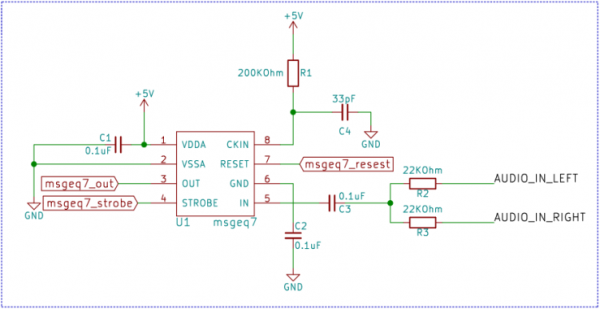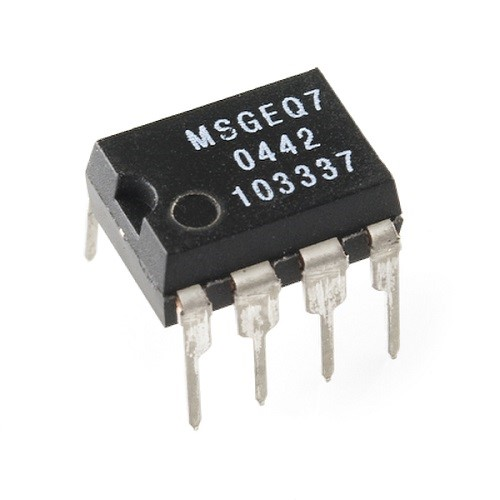• 쇼핑몰
• 커뮤니티
• 북마크

## 전기/전자#### [Equalizer]Graphic Equalizer

하나를하더라도최선을
2019.10.21 17:52 1,892 0 0 0
• - 첨부파일 : Graphic Equalizer.zip (383.0K) - 다운로드

## 본문# MSGEQ7 Interfacing with AVR

The MSGEQ7 is a 7 band graphic equalizer. What it does is detect the amplitudes of certain frequencies and gives an DC voltage output. The frequencies in question are 63Hz, 160Hz, 400Hz, 1kHz, 2.5kHz, 6.25kHz and 16kHz. Now going through the datasheet of MSGEQ7 we find that it will work with 3.3V or 5V, which is good as our ATMEGA8A is a 5V device. Next we need to build the schematic for the MSGEQ7 IC.

# Hardware for MSGEQ7

We only need a few parts to get MSGEQ7 working. We need a 200KΩ resistor and a 33pF ceramic capacitor at pin number 8. These two will set the clocking frequency for the MSGEQ7. A 0.1uF capacitor from pin 6 to ground and another from pin 1 to ground for noise protection. A pair of 22KΩ resistors one for each channel in series with a 0.01uF capacitor tied to the input pin number 5.

Fig 1. MSGEQ7 Connection Diagram

# Protocol for MSGEQ7

The MSGEQ7 has two control pins RESET and STROBE. After every RESET pulse the multiplexer inside the IC is reset and after every STROBE pulse MSGEQ7 will send the DC voltage corresponding to the frequency on the DC OUT pin. For the First STROBE pulse after RESET the DC OUTPUT voltage will correspond to the first frequency i.e. 63Hz. The next STROBE will correspond with the next frequency i.e. 160Hz and so on. After the 7th STROBE pulse the multiplexer will come back to 63Hz.

Fig 2. Timing Diagram

# Schematic

Fig 3. Atmega8 Interfacing With MSGEQ7

# Software

main.c

 1 2 3 4 5 6 7 8 9 10 11 12 13 14 15 16 17 18 19 20 21 22 23 24 25 26 27 28 29 30 31 32 33 34 35 36 37 38 39 40 41 42 43 44 45 46 47 48 49 50 51 52 53 54 55 56 57 58 59 60 61 62 63 64 65 66 67 68 69 70 71 72 73 74 75 76 77 `#include `   `#include "msgeq7.h"`   ```//Three LEDSs connected to PWM Outputs``` ```#define SET_LED1_BRIGHTNESS(x) (OCR2=x)``` ```#define SET_LED2_BRIGHTNESS(x) (OCR1BL=x)``` ```#define SET_LED3_BRIGHTNESS(x) (OCR1AL=x)```       `void` `IO_init(void);` `void` `TIMER_init(void);` `void` `ADC_init(void);` `unsigned char` `ReadAnalog(void);`   `int` `main(void)` `{` `unsigned char` `result;` `IO_init();` `TIMER_init();` `ADC_init();` ```/* Replace with your application code */``` `msgeq7_reset();` `while` `(1)` `{` `msgeq7_getValues((char` `*)&result);` `SET_LED1_BRIGHTNESS(result);` `SET_LED2_BRIGHTNESS(result);` `SET_LED3_BRIGHTNESS(result);` `}` `}`   `void` `IO_init(void){` `//PORTB as output` `DDRB=0XFF;`   `//PORTB as current source` `PORTB=0XFF;`   `//PORTD pins D7 and D4 as output` `DDRD|=(1<

msgeq7.h

 1 2 3 4 5 6 7 8 9 10 11 12 13 14 15 16 `#ifndef INCFILE1_H_` `#define INCFILE1_H_`   `#define STROBE_PORT PORTD` `#define STROBE_PIN PORTD4`   `#define RESET_PORT PORTD` `#define RESET_PIN PORTD7`   `void` `msgeq7_reset(void);` `void` `msgeq7_getValues(char` `* const);`         `#endif /* INCFILE1_H_ */`

msgeq7.c

 1 2 3 4 5 6 7 8 9 10 11 12 13 14 15 16 17 18 19 20 21 22 23 24 25 26 27 28 29 30 `#define F_CPU 16000000` `#include ` `#include ` `#include "msgeq7.h"` `unsigned char` `ReadAnalog(void);`   `void` `msgeq7_reset(){` ```STROBE_PORT|=(1 << STROBE_PIN); //make sure that STORBE pin is high before RESET``` `_delay_us(100);`   `RESET_PORT|=(1 << RESET_PIN);` `_delay_us(100);`   ```RESET_PORT &= ~(1 << RESET_PIN);``` `_delay_us(100);`   `}` `void` `msgeq7_getValues(char` `* const` `result){` `unsigned char` `i=0;` `for(i=0;i<7;i++){` ```STROBE_PORT&= ~(1 << STROBE_PIN);``` `_delay_us(100);` `result[i]=ReadAnalog();`   `if(result[i]<60)` `result[i]=0;` ```STROBE_PORT|=(1 << STROBE_PIN);``` `_delay_us(100);` `}` `}`

0 0
로그인 후 추천 또는 비추천하실 수 있습니다.

#### 댓글목록 0

등록된 댓글이 없습니다.
전체 3 건 - 1 페이지
제목
하나를하더라도최선을 3,587 0 0 2019.12.10
하나를하더라도최선을 3,232 0 0 2019.12.17
하나를하더라도최선을 1,893 0 0 2019.10.21